# CBSE Class 10 Statistics Sure Shot Questions Set A

## Study Material for Class 10 Statistics Chapter 14 Statistics

Class 10 Statistics students should refer to the following Pdf for Chapter 14 Statistics in standard 10. These notes and test paper with questions and answers for Grade 10 Statistics will be very useful for exams and help you to score good marks

### Class 10 Statistics Chapter 14 Statistics

CBSE Class 10 Statistics Sure Shot Questions Set A. There are many more useful educational material which the students can download in pdf format and use them for studies. Study material like concept maps, important and sure shot question banks, quick to learn flash cards, flow charts, mind maps, teacher notes, important formulas, past examinations question bank, important concepts taught by teachers. Students can download these useful educational material free and use them to get better marks in examinations.  Also refer to other worksheets for the same chapter and other subjects too. Use them for better understanding of the subjects.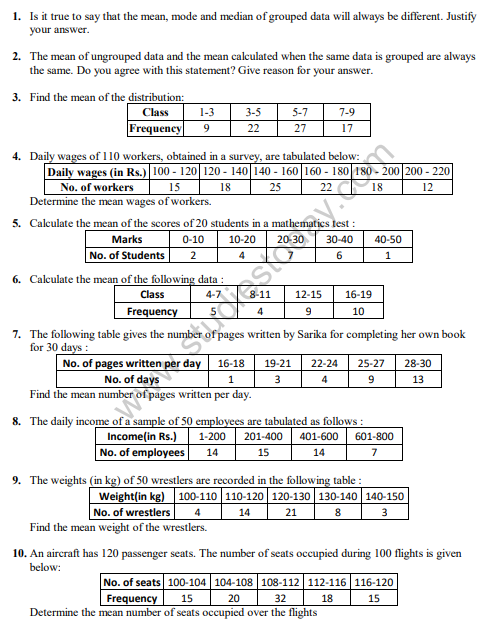More MCQs for NCERT Class 10 Mathematics Statistics.......

1. Consider the following table: The mode of the above data is(A) 23.5

(B) 24

(C) 24.4

(D) 25

2. The median and mode of a frequency distribution are 26 and 29 respectively. Then, the mean is

(A) 27.5

(B) 24.5

(C) 28.4

(D) 25.8

3. For a symmetrical frequency distribution, we have

(A) mean < mode < median

(B) mean > mode > median

(C) mean = mode = median

(D) mode = 1/2 (mean + median)

4. Look at the cumulative frequency distribution table given below: Number of families having income range 20000 to 25000 is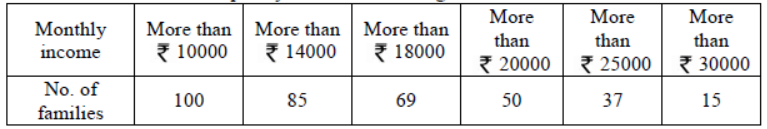(A) 19

(B) 16

(C) 13

(D) 22

5. If the mean of a data is 27 and its median is 33. Then, the mode is

(A) 30

(B) 43

(C) 45

(D) 47

6. Which measure of central tendency is obtained graphically as the x-coordinate of the point of intersection of the two ogives?

(A) Mean

(B) Median

(C) Mode

(D) None of these

7. For the following distribution: The sum of the lower limits of the median class and the modal class is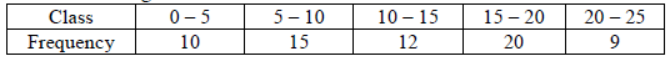(A) 15

(B) 25

(C) 30

(D) 35

8. Consider the following frequency distribution: The upper limit of the median class is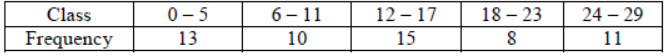(A) 16.5

(B) 18.5

(C) 18

(D) 17

9. For finding the mean by using the formula x  = A + h (∑fiui /∑fi) , we have ui = ?

(A) (A - xi )/h

(B) (xi - A) /h

(C) (A + xi ) /h

(D) h(xi - A)

10. While computing the mean of the grouped data, we assume that the frequencies are

(A) evenly distributed over the classes

(B) centred at the class marks of the classes

(C) centred at the lower limits of the classes

(D) centred at the upper limits of the classes

11. Consider the frequency distribution of the heights of 60 students of a classThe sum of the lower limit of the modal class and the upper limit of the median class is

(A) 310

(B) 315

(C) 320

(D) 330

12. Mode = ?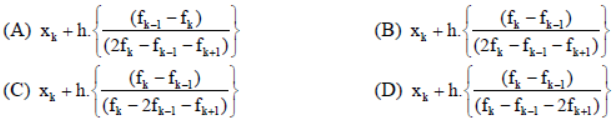13. If the mean and median of a set of numbers are 8.9 and 9 respectively, then the mode will be
(A) 7.2

(B) 8.2

(C) 9.2

(D) 10.2

14. Look at the frequency distribution table given below: The median of the above distribution is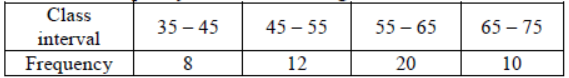(A) 56.5

(B) 57.5

(C) 58.5

(D) 59